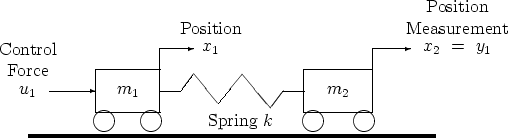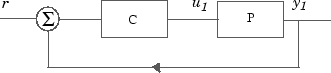## System with Uncertain Parameters

As an example of a closed-loop system with uncertain parameters, consider the two-cart "ACC Benchmark" system  consisting of two frictionless carts connected by a spring shown as follows.

ACC Benchmark ProblemThe system has the block diagram model shown below, where the individual carts have the respective transfer functions.

`$\begin{array}{l}{G}_{1}\left(s\right)=\frac{1}{{m}_{1}{s}^{2}}\\ {G}_{2}\left(s\right)=\frac{1}{{m}_{2}{s}^{2}}.\end{array}$`

The parameters m1, m2, and k are uncertain, equal to one plus or minus 20%:

```m1 = 1 ± 0.2 m2 = 1 ± 0.2 k = 1 ± 0.2 ```

"ACC Benchmark" Two-Cart System Block Diagram y1 = P(s) u1The upper dashed-line block has transfer function matrix F(s):

`$F\left(s\right)=\left[\begin{array}{c}0\\ {G}_{1}\left(s\right)\end{array}\right]\left[\begin{array}{cc}1& -1\end{array}\right]+\left[\begin{array}{c}1\\ -1\end{array}\right]\left[\begin{array}{cc}0& {G}_{2}\left(s\right)\end{array}\right].$`

This code builds the uncertain system model `P` shown above:

```m1 = ureal('m1',1,'percent',20); m2 = ureal('m2',1,'percent',20); k = ureal('k',1,'percent',20); s = zpk('s'); G1 = ss(1/s^2)/m1; G2 = ss(1/s^2)/m2; F = [0;G1]*[1 -1]+[1;-1]*[0,G2]; P = lft(F,k);```

The variable `P` is a SISO uncertain state-space (USS) object with four states and three uncertain parameters, `m1`, `m2`, and `k`. You can recover the nominal plant with the command:

`zpk(P.nominal)`
```ans = 1 ------------- s^2 (s^2 + 2) Continuous-time zero/pole/gain model. ```

If the uncertain model P(s) has LTI negative feedback controller

`$C\left(s\right)=\frac{100{\left(s+1\right)}^{3}}{{\left(0.001s+1\right)}^{3}}$`then you can form the controller and the closed-loop system y1 = T(s) u1 and view the closed-loop system's step response on the time interval from t=0 to t=0.1 for a Monte Carlo random sample of five combinations of the three uncertain parameters `k`, `m1`, and `m2` using this code:

```C=100*ss((s+1)/(.001*s+1))^3; % LTI controller T=feedback(P*C,1); % closed-loop uncertain system step(usample(T,5),.1);```